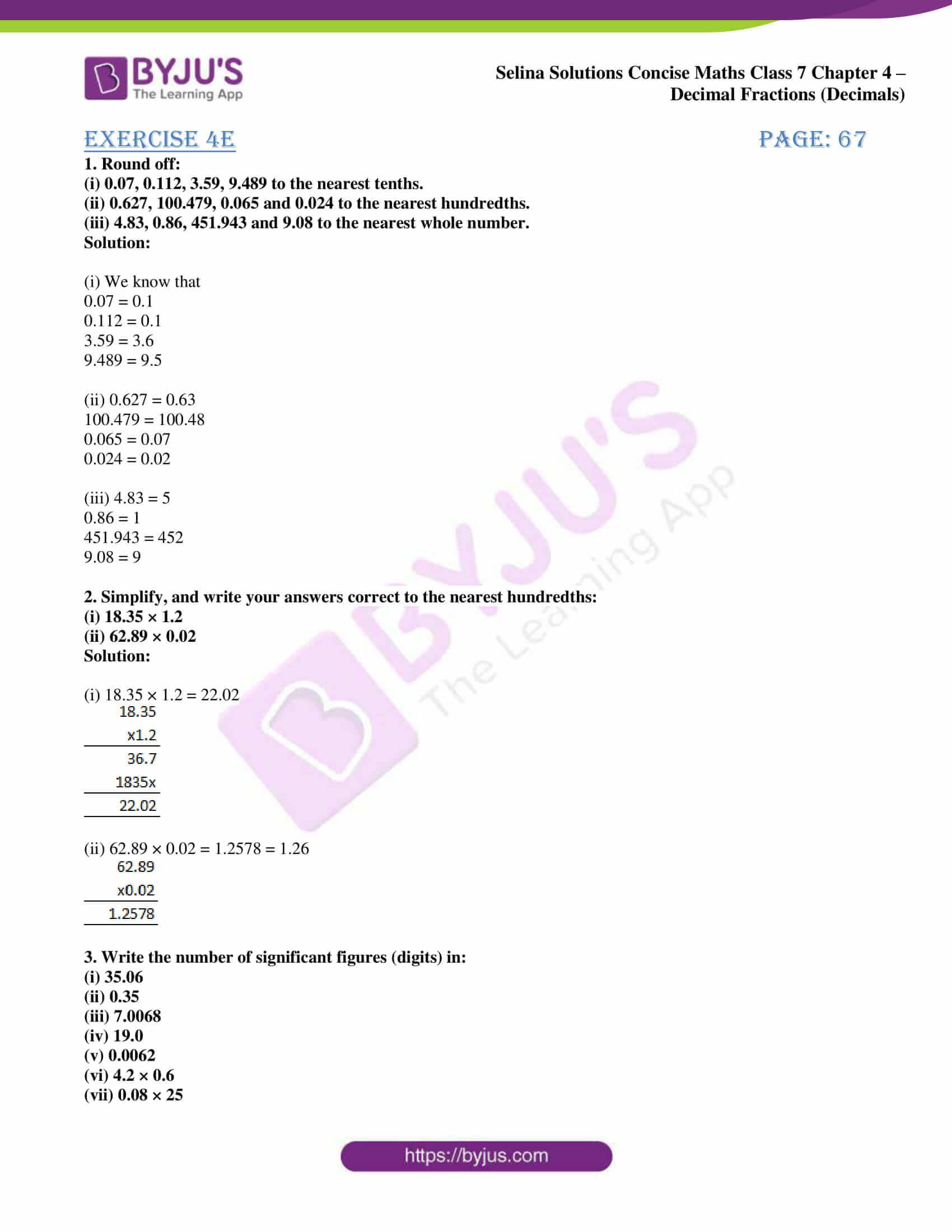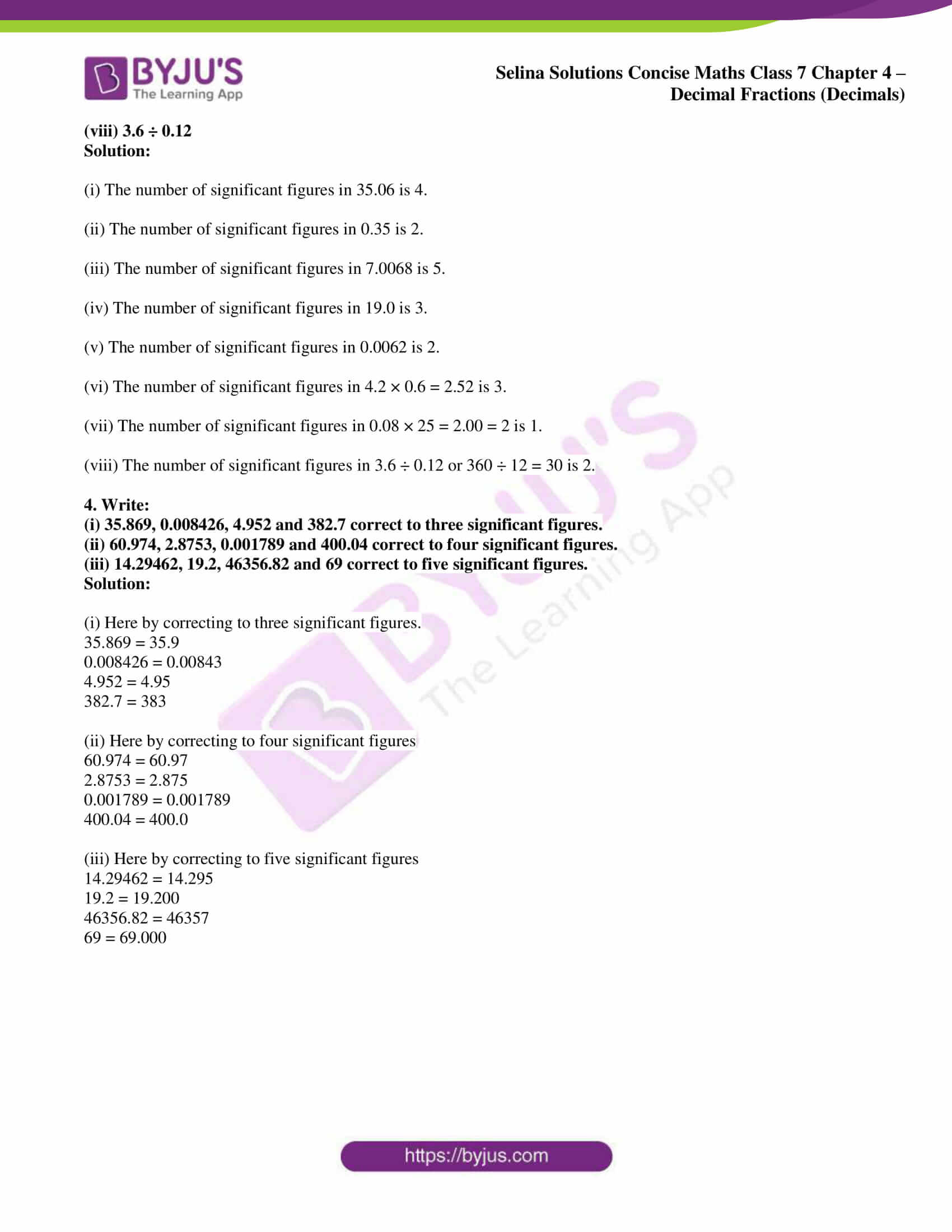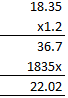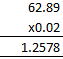# Selina Solutions Concise Maths Class 7 Chapter 4: Decimal Fractions (Decimals) Exercise 4E

Selina Solutions Concise Maths Class 7 Chapter 4 Decimal Fractions (Decimals) Exercise 4E helps students understand rounding-off of decimal numbers. The teachers at BYJU’S have created the solutions after conducting vast research on each concept. The solutions are available in PDF format and the students can download them, based on their requirements. Significant figures is another concept which is discussed under this exercise. Selina Solutions Concise Maths Class 7 Chapter 4 Decimal Fractions (Decimals) Exercise 4E, PDF links are given below for free download.

## Selina Solutions Concise Maths Class 7 Chapter 4: Decimal Fractions (Decimals) Exercise 4E Download PDF### Access Selina Solutions Concise Maths Class 7 Chapter 4: Decimal Fractions (Decimals) Exercise 4E

1. Round off:

(i) 0.07, 0.112, 3.59, 9.489 to the nearest tenths.

(ii) 0.627, 100.479, 0.065 and 0.024 to the nearest hundredths.

(iii) 4.83, 0.86, 451.943 and 9.08 to the nearest whole number.

Solution:

(i) We know that

0.07 = 0.1

0.112 = 0.1

3.59 = 3.6

9.489 = 9.5

(ii) 0.627 = 0.63

100.479 = 100.48

0.065 = 0.07

0.024 = 0.02

(iii) 4.83 = 5

0.86 = 1

451.943 = 452

9.08 = 9

(i) 18.35 × 1.2

(ii) 62.89 × 0.02

Solution:

(i) 18.35 × 1.2 = 22.02(ii) 62.89 × 0.02 = 1.2578 = 1.263. Write the number of significant figures (digits) in:

(i) 35.06

(ii) 0.35

(iii) 7.0068

(iv) 19.0

(v) 0.0062

(vi) 4.2 × 0.6

(vii) 0.08 × 25

(viii) 3.6 ÷ 0.12

Solution:

(i) The number of significant figures in 35.06 is 4.

(ii) The number of significant figures in 0.35 is 2.

(iii) The number of significant figures in 7.0068 is 5.

(iv) The number of significant figures in 19.0 is 3.

(v) The number of significant figures in 0.0062 is 2.

(vi) The number of significant figures in 4.2 × 0.6 = 2.52 is 3.

(vii) The number of significant figures in 0.08 × 25 = 2.00 = 2 is 1.

(viii) The number of significant figures in 3.6 ÷ 0.12 or 360 ÷ 12 = 30 is 2.

4. Write:

(i) 35.869, 0.008426, 4.952 and 382.7 correct to three significant figures.

(ii) 60.974, 2.8753, 0.001789 and 400.04 correct to four significant figures.

(iii) 14.29462, 19.2, 46356.82 and 69 correct to five significant figures.

Solution:

(i) Here by correcting to three significant figures.

35.869 = 35.9

0.008426 = 0.00843

4.952 = 4.95

382.7 = 383

(ii) Here by correcting to four significant figures

60.974 = 60.97

2.8753 = 2.875

0.001789 = 0.001789

400.04 = 400.0

(iii) Here by correcting to five significant figures

14.29462 = 14.295

19.2 = 19.200

46356.82 = 46357

69 = 69.000